# 《安家》热播，我用Python对北京房价进行了分析，结果……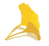id：交易编号

followers：关注人数

totalPrice：总价

price：单价

square：面积

livingRoom：卧室数量

drawingRoom：客厅数量

kitchen：厨房数量

bathRoom：浴室数量

floor：楼层

buildingType：建筑类型

buildingStructure：建筑结构

elevator：有无电梯

fiveYearsProperty：是否满五年

subway：有无地铁

disrict：所在区域

communityAverage：小区均价

1. 市场近几年的整体价格趋势是什么情况？

2. 房源在不同价格区间的大致分布状态是什么样子的？

1. 通过不同时间的交易数量可以大致判断有没有明显的交易淡旺季？

2. 通过关注人数可以了解哪些房源比较受欢迎，然后观察一下他们的价格及其他特征。

import pandas as pd
import numpy as np
import matplotlib as plt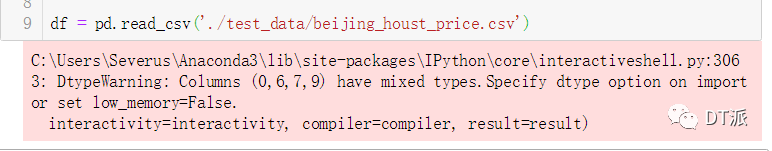df = pd.read_csv('./test_data/beijing_houst_price.csv',dtype={'id':'str', 'tradeTime':'str','livingRoom':'str','drawingRoom':'str','bathRoom':'str'})


df.shape
df.describe()
df.count()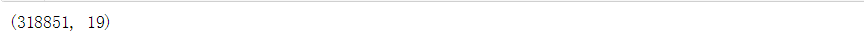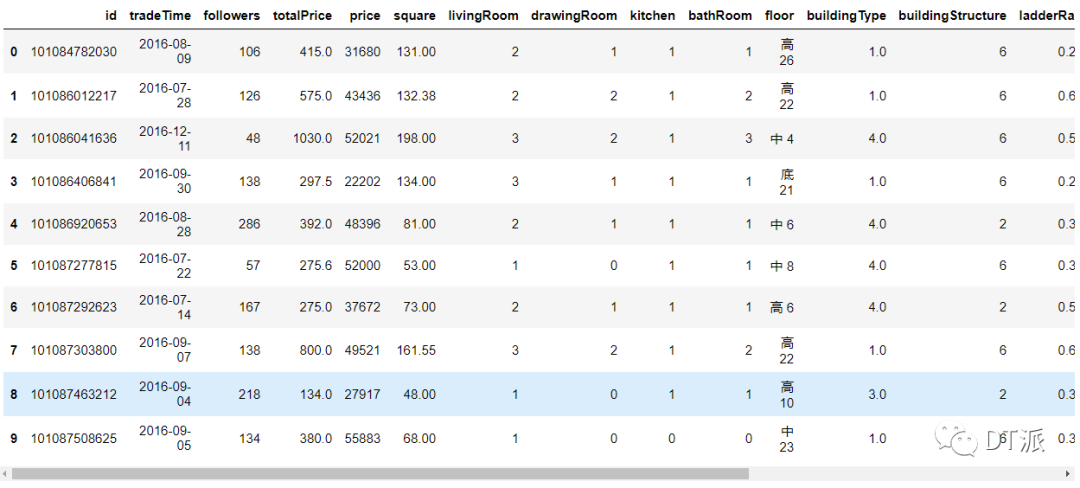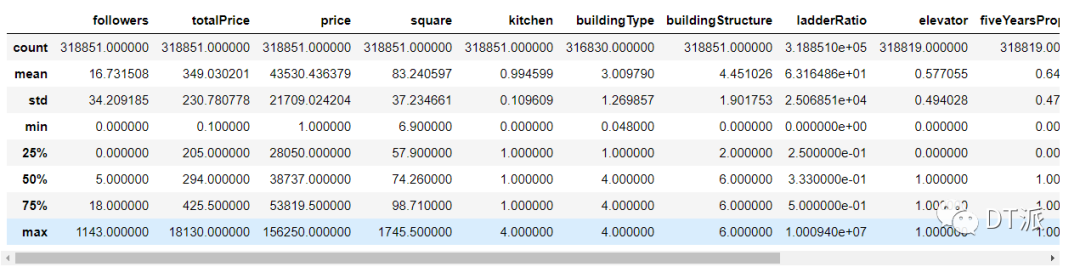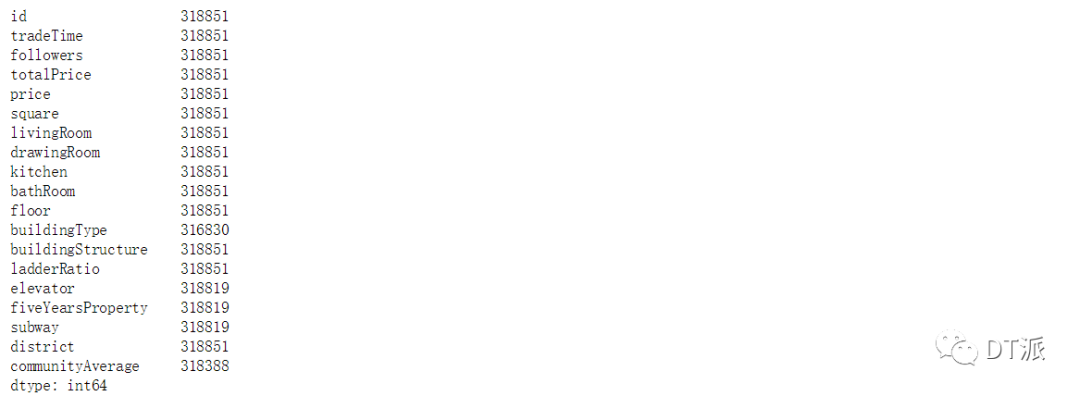count--计算每列中非空数值的数量

mean--返回每列的平均值

std--返回每列的标准差

min--返回每列的最小值

max--返回每列的最大值

（25%，50%，75%）--统计学四分位数，其中50%对应中位数

df[df['id'].duplicated()]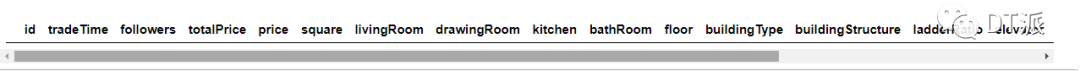df['tradeTime'] = pd.to_datetime(df['tradeTime'])
# df.dtypes    # 可以查看当前的数据类型


df['tradeTime'].dt.year.value_counts()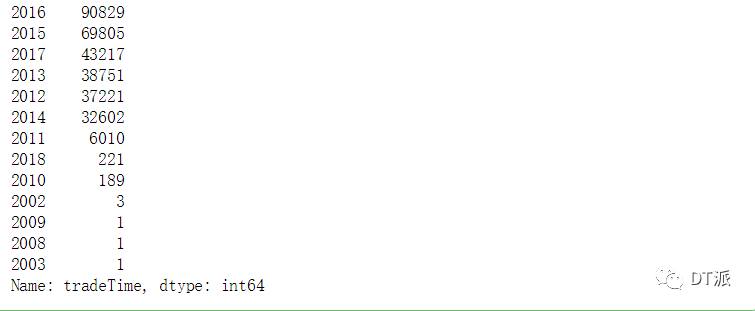df.drop(df[df['tradeTime'].dt.year < 2012].index, inplace=True)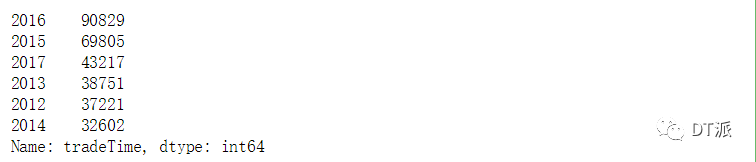df[df['totalPrice'] < 50]
df[df['totalPrice'] < 100]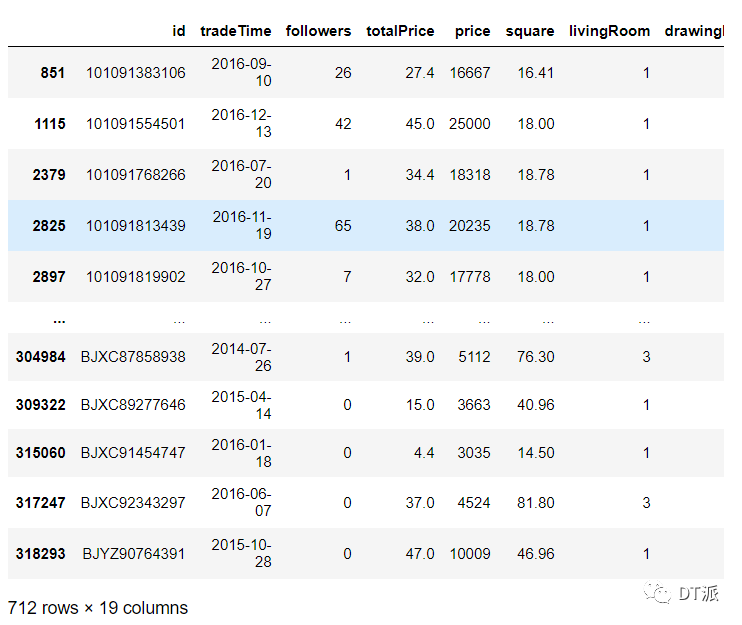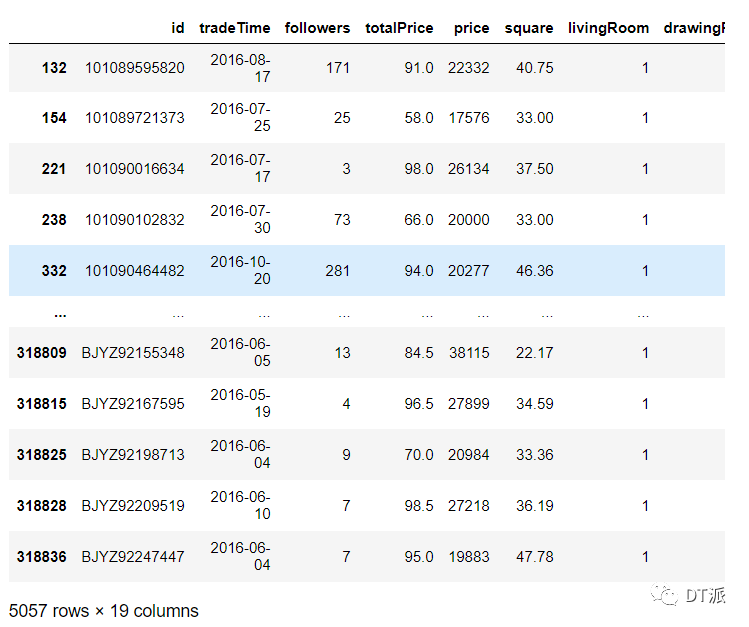df.drop(df[df['totalPrice'] < 100].index, inplace=True)
df.drop(df[df['price'] < 10000].index, inplace=True)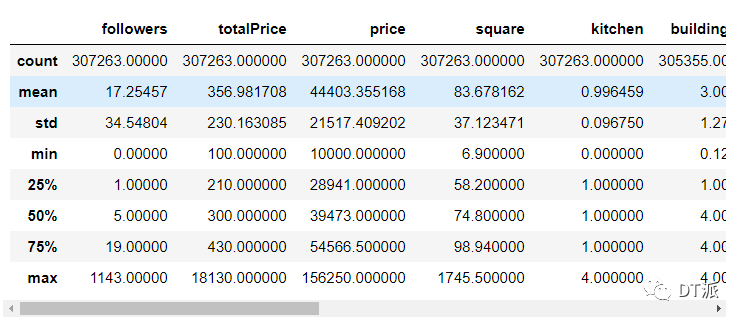df.drop(df[df['communityAverage'].isnull()].index, inplace=True)


communityAverage最大值和最小值合理，不过有空值，大约只有400条数据，这里我们直接删掉（也可以选择填充），最后再看一下square列，值都在合理的范围内，并且也没有空值，现在，数据清洗就已经完成了，接下来可以开始分析。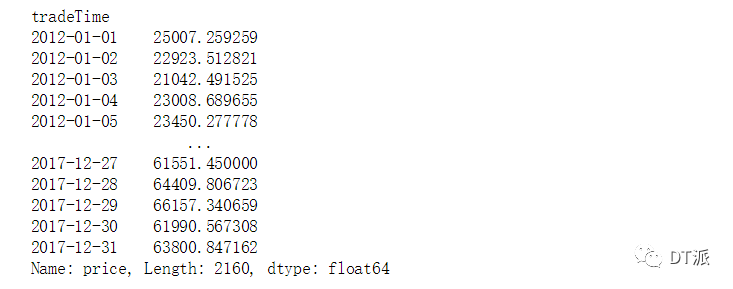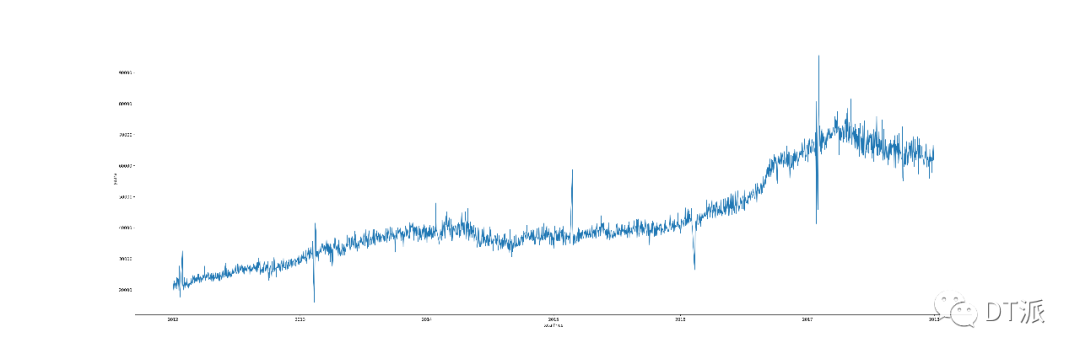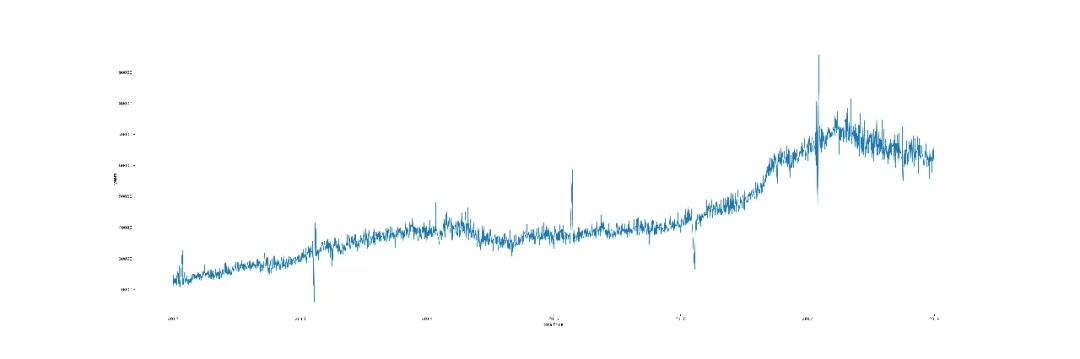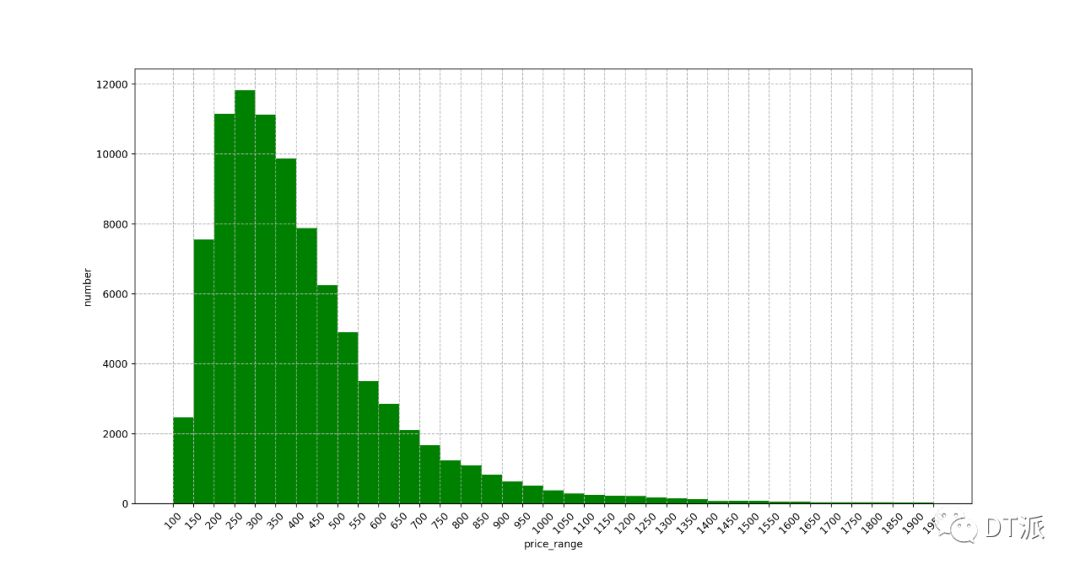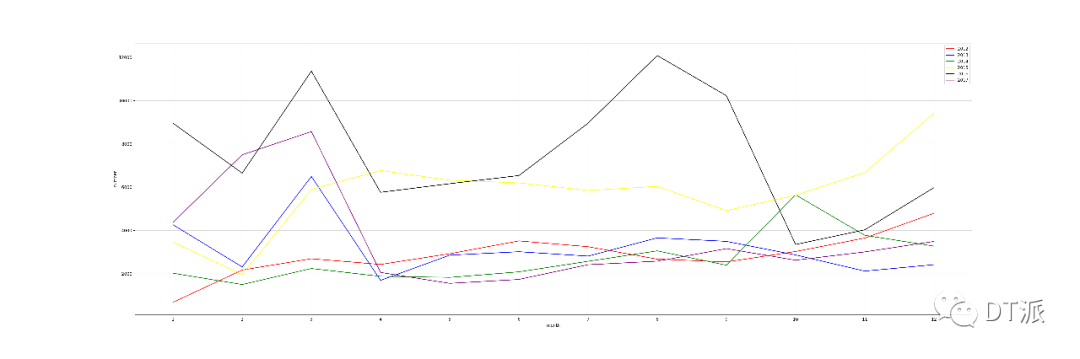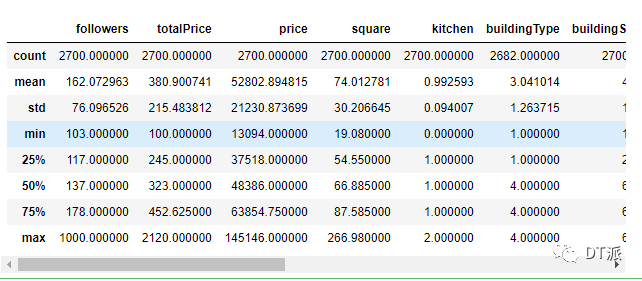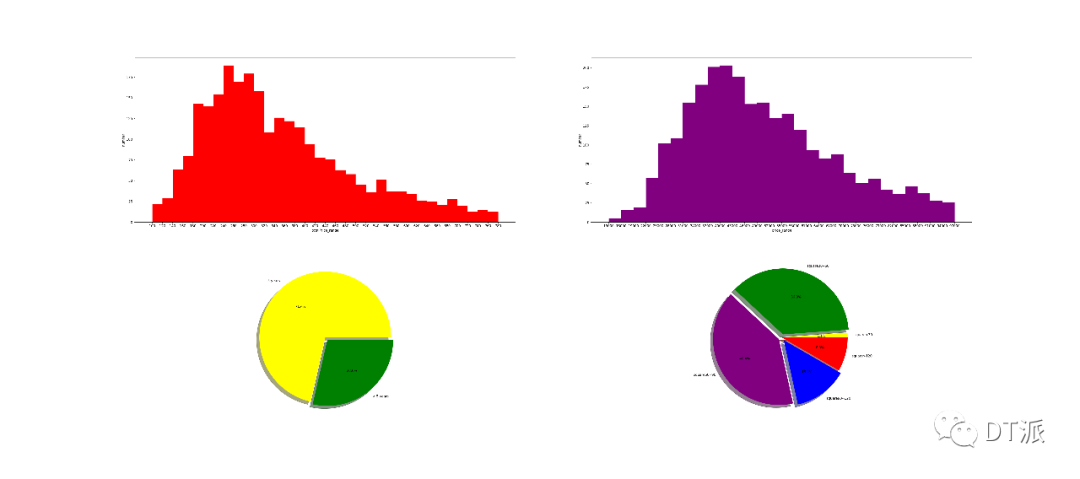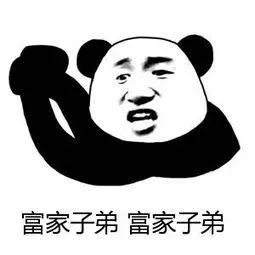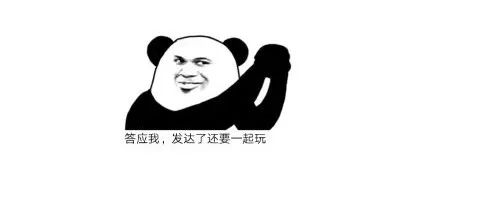©️2019 CSDN 皮肤主题: 大白 设计师: CSDN官方博客